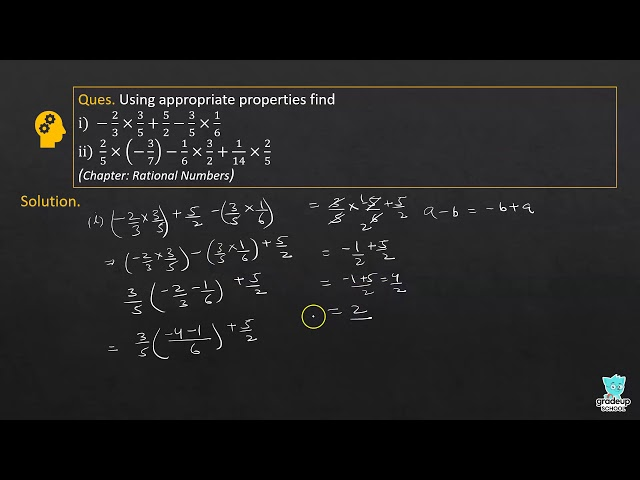# NCERT Solutions for Class 8 Maths Chapter 1 - Rational Numbers

Share

Goprep offers students with NCERT Mathematics Solutions for Class 8 Chapter 1 - Rational Numbers solved by expert subject teachers which help you in revising the complete syllabus of the exam and scoring more marks in the exam. Prepared in accordance with the CBSE guidelines, these NCERT Solutions provide students with a detailed and explained answers to the questions in a systematic format.

NCERT Mathematics Solutions Chapter 1- Rational Numbers deals with important topics like Number Systems, Rational Numbers, Its properties and Applications, Study of Algebraic Operations, etc. Further, introduction to Rational Numbers, Properties of Rational Numbers, Closure, Commutativity, The Role of Zero, Associativity, The Role of One, Negative of a Number, Reciprocal, Distributivity of Multiplication over Addition over Rational Numbers, Representation of Rational Numbers on Number Line, etc. So, with the help of these solved questions of the NCERT textbook, understanding the concepts of these topics become easy for the students.

## NCERT Solutions for Class 8 Maths Chapter 1 - Rational Numbers

Exercises 1.1
• Exercises 1.1
• Exercises 1.2
Class 8th|NCERT - MathematicsChapter 1 - Rational NumbersQ. 10

Write.

See More (1)
NCERT - MathematicsClass 8th , NCERT
 Chapter 1 - Rational Numbers Chapter 2 - Linear Equations in One Variable Chapter 3 - Understanding Quadrilaterals Chapter 4 - Practical Geometry Chapter 5 - Data Handling Chapter 6 - Squares and Square Roots Chapter 7 - Cubes and Cube Roots Chapter 8 - Comparing Quantities Chapter 9 - Algebraic Expressions and Identities Chapter 10 - Visualising Solid Shapes Chapter 11 - Mensuration Chapter 12 - Exponents and Powers Chapter 13 - Direct and Inverse Proportions Chapter 14 - Factorisation Chapter 15 - Introduction to Graphs Chapter 16 - Playing with Numbers